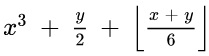# Quiz #1 for October 6, 2020

Please use your Python system (IDLE or something else) to compute the following. Be prepared to show your work!

1. If x = 3 and y = 1, what is:Answer: The Python expression is: x**3 + y/2 + (x+y)//6. This evaluates to
3**3 + 18/2 + (3 + 18) / 6 = 27 + 9.0 + 21 // 6 = 27 + 9 + 3 = 39
Note the second division is integer division, which is the floor function.

2. What is the remainder of 2,347,500,001 when it is divided by 6,345?
Answer: You never put commas in numbers in Python. So the Python expression to evaluate is
2347500001 % 6345
which gives 2281.

3. True or false: The value of 2 + 3 % 2 is 1, because 2 + 3 is 5, and 5 % 2 is 1.
Answer: False. The modulo or remainder operator (%) has higher precedence than the addition (+) operstor, so you do the % first. This gives 2 + 3 % 2 = 2 + 1 = 3.Matt Bishop Office: 2209 Watershed Sciences Phone: +1 (530) 752-8060 Email: mabishop@ucdavis.edu
MHI 289I, Programming for Health Informatics
Version of October 6, 2020 at 2:19PM